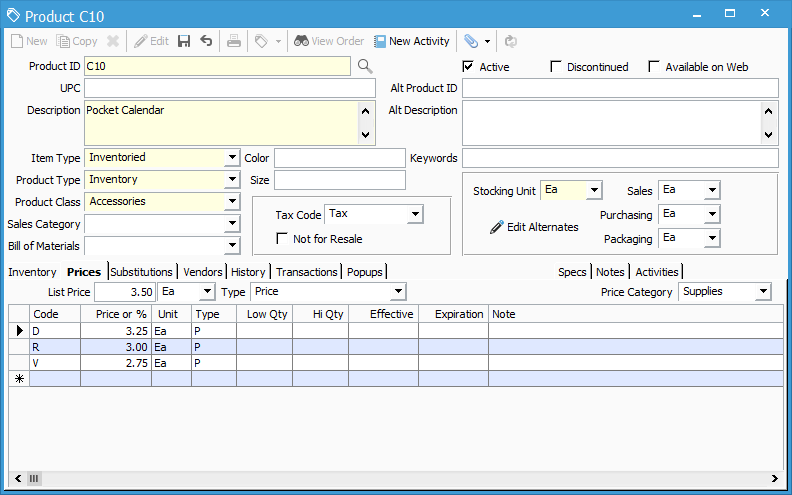# Product Information Window – Prices Tab

This tab is a powerful feature in Acctivate that allows products to be priced in numerous ways. Special prices may be established for certain types of customers by Price Code, or the list price may be configured based on the cost using one of five formulas.List Price Type: Each product may specify a List Price Type.

• P ( Price ): Sets the price to dollar amount listed in Price/Pct column. This overrides the existing list price for the product.
• C\$ ( Average Cost + Amount ): Charges the average cost for the product plus the dollar amount listed in the Price/Pct column. Average cost is the total value of a product divided by the quantity of the product.
• C% ( Average Cost + Percent ): Starts with the average cost for the product and adds a numerical percentage listed in the Price/Pct column. Average cost is the total value of a product divided by the quantity of the product.
• L\$ ( List Price + Amount ): Charges the list price for the product plus the dollar amount listed in the Price/Pct column.
• L% ( List Price – Percent ): Starts with the list price for the product and subtracts a numerical percentage listed in the Price/Pct column.
• M\$ ( Last Cost + Amount ): Sets price by using the last cost for the product and adds that dollar amount listed in the Price/Pct column. Positive numbers add to the last cost and negative numbers subtract from the last cost. Last cost is based on warehouse.
• M% ( Last Cost + Percent ): Starts with the last cost for a product receipt and adds the percentage listed in the Price/Pct column. Positive numbers add a percentage to the last cost and negative numbers subtract a percentage from the last cost. Last cost is based on warehouse.
• S\$ ( Management + Amount ): Charges the management cost for the product plus the dollar amount listed in the Price/Pct column. The management cost is located on the inventory tab of the product and is based on warehouse.
• S% ( Management + Percent ): Starts with the management cost for the product and adds a numerical percentage listed in the Price/Pct column. Positive numbers add a percentage to the management cost and negative numbers subtract a percentage from the management cost. The management cost is located on the inventory tab of the product and is based on warehouse.

List Price: Each product may specify a standard List Price. This is the default price that will populate on a Sales Order when the product is selected.

Unit: The unit of measure specified for the price (\$1.00 each and \$1.00 per lb can mean two different things in Acctivate) .

Price Category: Products may be priced by a Price Category that is shared with other products. In this shared pricing model, a common set of prices may be established for a particular Price Category.